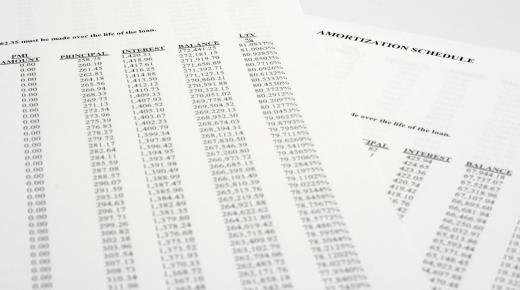Finance
Fact Checked

# What Is Simple Interest?

Carol Francois
Carol Francois

Simple interest is the value of money over a specific period of time. Interest is a mathematical calculation of the cost to borrow money or the amount earned from lending money. Simple interest is most commonly used for loans and investments.

The calculation for simple interest uses three items: principle, interest rate, and length of time. Principle is the total amount of money borrowed or invested. Interest rate is the percentage rate used to calculate the interest amount. The length of time is the same as the repayment period. The longer the loan is for, the more it will cost in interest.An amortization schedule is a table with the details of the amount of each payment allocated to principal and interest.

The formula to calculate simple interest is I = PRT. In this formula, "P" is the principle amount of the loan, "R" is the interest rate, which is expressed as a percentage value and "T" is the number of periods in time. If the time is provided in days, then simply create a fraction with the number of days as the numerator and 365 as the denominator.

Interest calculations are used for three reasons: to evaluate the cost of financing, to determine the amount owing, and to calculate the interest rate payment on an investment. When comparing two sources of financing, it is important to make sure that you are comparing the same details. Make sure that the periods and the length of the term are the same.

Write down the total amount being borrowed, as well as the rate and length of the term. Then calculate the interest rate and the amount of interest to be paid. Many states require all financing companies to provide this exact information when receiving a loan of any type. If the loan is open, the borrower can pay the principle to be paid off early with no penalty. This is the best way to reduce the cost of a loan.

When comparing investment opportunities, read the prospectus carefully to determine how the interest will be calculated and when it will be paid. Bonds, investment certificates, and treasury bills typically pay simple interest. The rate is based on a number of factors, including the bank standard interest rate, inflation, and alternate investment opportunities.

Investments in stocks, mutual funds or other items do not pay interest. Instead, these investments earn money by increasing in price during the time period between when the stock was purchased and when you want to sell it. Some investments pay dividends, which is a portion of the company profit distributed among shareholders. The amount and frequency of the dividend payout depends on the performance of the company and other factors.

## You might also Like